# Fisher linear discriminant and dataset transformation

Bob Fisher

The Fisher Linear Discriminant (FLD) gives a projection matrix W that reshapes the scatter of a data set to maximize class separability, defined as the ratio of the between-class scatter matrix to the within-class scatter matrix. This projection defines features that are optimally discriminating.

Let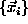be a set of N column vectors of dimension D. The mean of the dataset is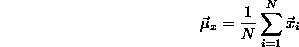There are K classes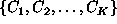. The mean of class k containing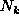members is: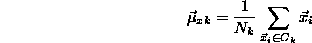The between class scatter matrix is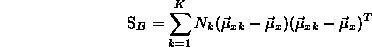The within class scatter matrix is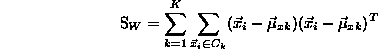The transformation matrix that repositions the data to be most separable is the matrix W that maximizes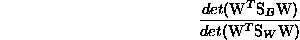Let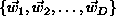be the generalized eigenvectors of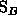and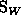. Then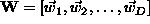. This gives a projection space of dimension D. A projection space of dimension d < D can be defined by using the generalized eigenvectors with the largest d eigenvalues to give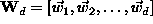. The projection of vector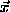into a subspace of dimension d is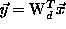.

The generalized eigenvectors are eigenvectors of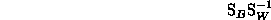Bob Fisher
Friday June 15 18:23:13 BST 2001